Question Pool Decimal Forms

# Decimal Forms - SAMAGRA Question Pool & Answers | Class 9 English Medium

Kerala Syllabus SAMAGRA SCERT SAMAGRA Question Pool for Class 9 English Medium Maths Decimal FormsQn 1.

Examine the method  of finding the decimal form of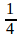=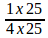=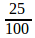=.25

a)In this method, Write  the decimal form of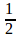,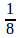?

b)+=  ..........?

Get Free Study Materials + 1 Week Free Trial of BrainsPrep Class 9 English Medium Tuition

Qn 2.

Split the following decimal forms as sum of the reciprocal of the powers of 10 as place values.

i) 0.85

ii) 0.304

iii) 0.016

Get Free Study Materials + 1 Week Free Trial of BrainsPrep Class 9 English Medium Tuition

Qn 3.

Find the decimal forms of the sums below

i)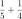ii)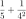Get Free Study Materials + 1 Week Free Trial of BrainsPrep Class 9 English Medium Tuition

Qn 4.

Write the decimal form

i)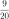ii)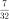Get Free Study Materials + 1 Week Free Trial of BrainsPrep Class 9 English Medium Tuition

Qn 5.

Write the denominator of the fraction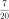as the product of prime factors.

ii)

Write the decimal form ofGet Free Study Materials + 1 Week Free Trial of BrainsPrep Class 9 English Medium Tuition

Qn 6.

i) Write the decimal form ofii) Write the fractional form of 0.625

iii) A two-digit number divided by another two-digitn number gives 4.625. What are the numbers?

iv) Write 4.625 as fraction with numerator a three-digit number and denominator two-digit number. Write more such fractions.

Get Free Study Materials + 1 Week Free Trial of BrainsPrep Class 9 English Medium Tuition

Qn 7.

i) Write the decimal form ofii) Write 0.375 as afraction.

iii) A two-digit number divided by another two-digit number gives 5.375. What are the numbers?

iv) Write 5.375 as a fraction with numerator a three-digit number and denominator two-digit number. Write more such fractions.